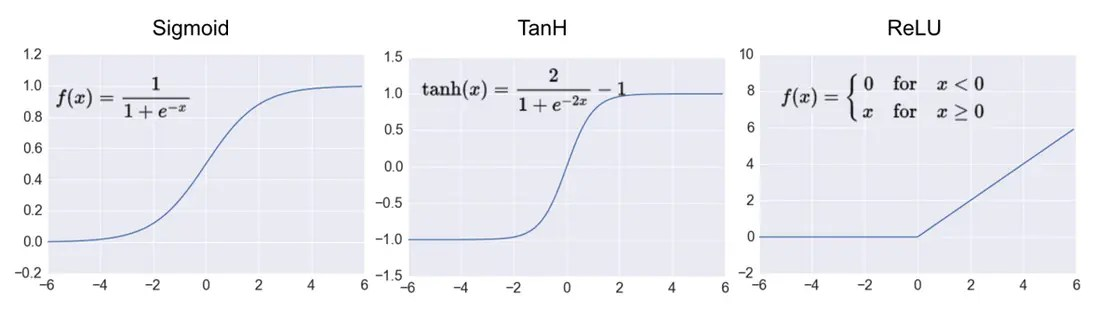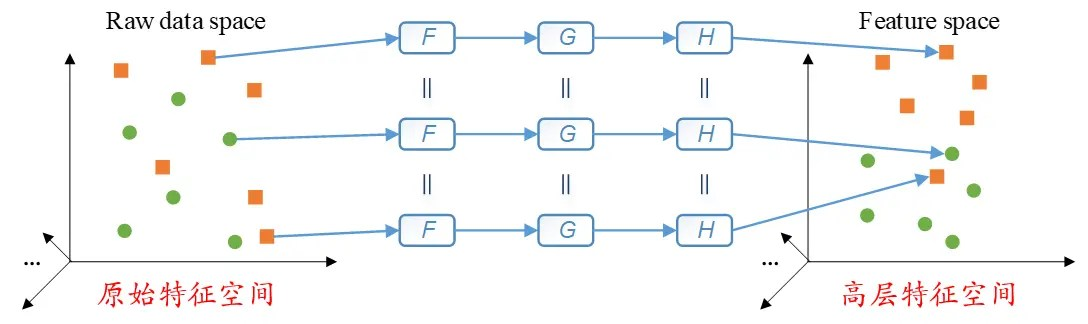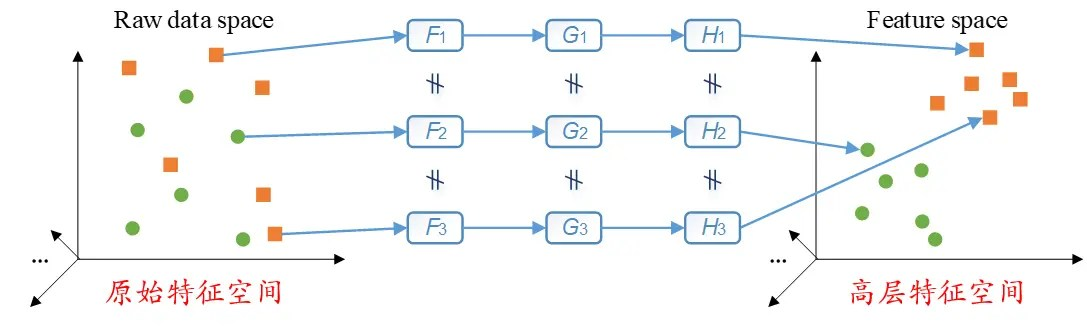# 【Harbin Institute of Technology】 Dynamic ReLU: the basic principle of adaptive parameterized ReLU

Posted May 25, 20205 min read

Adaptive parameterized ReLU is a dynamic activation function, which is not "equal to all", submitted to IEEE Transactions on Industrial Electronics on May 3, 2019, accepted on January 24, 2020, Published on the IEEE's official website on February 13, 2020.

Based on a review of the traditional activation function and attention mechanism, this article interprets a dynamic activation function under the attention mechanism, namely Adaptive Parametric Rectifier Linear Unit(APReLU). help.

#### 1 . The traditional activation function is static

Activation function is an important part of modern artificial neural network, and its function is to realize the nonlinearity of artificial neural network. Let's first introduce some of the most common activation functions, namely Sigmoid activation function, Tanh activation function and ReLU activation function, as shown below:The gradient range of Sigmoid activation function and Tanh activation function are(0,1) and(-1,1), respectively. When there are many layers, the artificial neural network may encounter the problem of gradient disappearance. The gradient of the ReLU activation function is either zero or one, which can well avoid the problems of gradient disappearance and gradient explosion, so it has been widely used in recent years.

However, the ReLU activation function still has a flaw. If during the training process of the artificial neural network, all the features are less than zero, then the output of the ReLU activation function is all zero. At this time, the training failed. In order to avoid this situation, some scholars have proposed the leaky ReLU activation function, instead of setting features less than zero to zero, but multiplying features less than zero by a small coefficient, such as 0.1 and 0.01.

In leaky ReLU, the value of this coefficient is set manually. However, the manually set coefficients are not necessarily the best, so He Kaiming et al. Proposed the Parametric ReLU activation function(parametric ReLU activation function, PReLU activation function). Set this coefficient to a parameter that can be trained. During the training process, gradient descent method is used for training together with other parameters. However, the PReLU activation function has a characteristic:once the training process is completed, the coefficient in the PReLU activation function becomes a fixed value. In other words, for all test samples, the value of this coefficient in the PReLU activation function is the same.Here we have roughly introduced several commonly used activation functions. What's wrong with these activation functions? We can think about it. If an artificial neural network uses one of the above activation functions or a combination of the above activation functions, then after the training is completed, when the artificial neural network is applied to the test samples, all the test samples The nonlinear transformation used is the same, that is, static. In other words, all test samples will undergo the same nonlinear transformation. This is actually a more rigid way.

As shown in the following figure, if we represent the original feature space with the scatter diagram on the left, and the high-level feature space learned by the artificial neural network with the scatter diagram on the right, the small dots and small squares in the scatter diagram represent two For different types of samples, F, G, and H represent nonlinear functions. Then these samples are transformed from the original feature space to the high-level feature space through the same nonlinear function. In other words, the "=" in the picture means that for these samples, the nonlinear transformation they undergo is exactly the same.So, can we set the parameters of the activation function for each sample separately according to the characteristics of each sample, so that each sample undergoes a different dynamic nonlinear transformation? The APReLU activation function to be introduced later in this article does just that.

#### 2 . Attention mechanism

The APReLU activation function to be introduced in this article draws on the classic Squeeze-and-Excitation Network(SENet), and SENet is a very classic, deep learning method under the attention mechanism. The basic principle of SENet is shown below:Here is an introduction to the ideas contained in SEnet. For many samples, the importance of each feature channel in the feature map is likely to be different. For example, feature channel 1 of sample A is very important and feature channel 2 is not important; feature channel 1 of sample B is not important and feature channel 2 is very important; then at this time, for sample A, we should focus on features Channel 1(that is, give feature channel 1 a higher weight); conversely, for sample B, we should focus on feature channel 2(that is, give feature channel 2 a higher weight).

In order to achieve this goal, Senet learned a set of weight coefficients through a small fully connected network, and weighted each channel of the original feature map. In this way, each sample(including training samples and test samples) has its own unique set of weights, which are used to weight each feature channel. This is actually an attention mechanism, that is, to pay attention to important feature channels, and then give them a higher weight.

#### 3 . Adaptive parameterized correction linear unit(APReLU) activation function

APReLU activation function, in essence, is the integration of SENet and PReLU activation function. In SEnet, the weights learned by the small fully connected network are the weights used for each feature channel. The APReLU activation function also obtains weights through a small fully connected network, and then uses this set of weights as the coefficients in the PReLU activation function, that is, the weight of the negative part. The basic principle of APReLU activation function is shown in the figure below.We can see that in the APReLU activation function, the form of its nonlinear transformation is exactly the same as the PReLU activation function. The only difference is that the weight coefficients for negative features in the APReLU activation function are learned through a small fully connected network. When the artificial neural network uses the APReLU activation function, each sample can have its own unique weight coefficient, that is, a unique nonlinear transformation(as shown in the following figure). At the same time, the input feature map and output feature map of the APReLU activation function have the same size, which means that APReLU can be easily embedded into existing deep learning algorithms.In summary, the APReLU activation function allows each sample to have its own unique set of nonlinear transformations, providing a more flexible method of dynamic nonlinear transformation, with the potential to improve the accuracy of pattern recognition.

references:
Zhao M, Zhong S, Fu X, et al. Deep residual networks with adaptively parametric rectifier linear units for fault diagnosis \ [J ]. IEEE Transactions on Industrial Electronics, 2020, DOI:10.1109/TIE.2020.2972458, Date of Publication: 13 February 2020